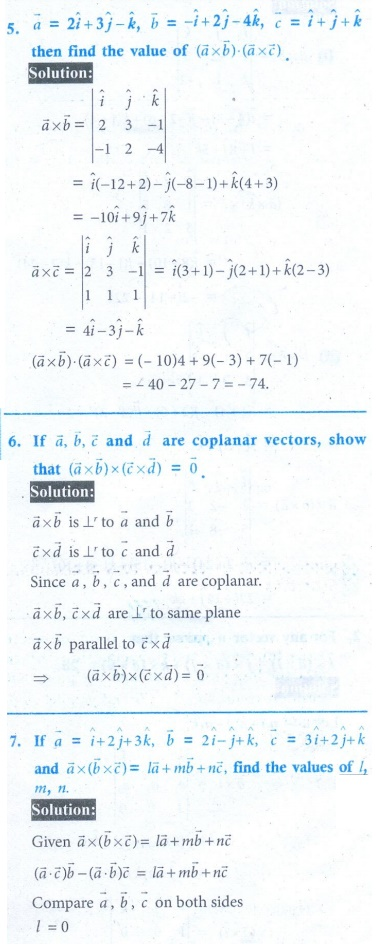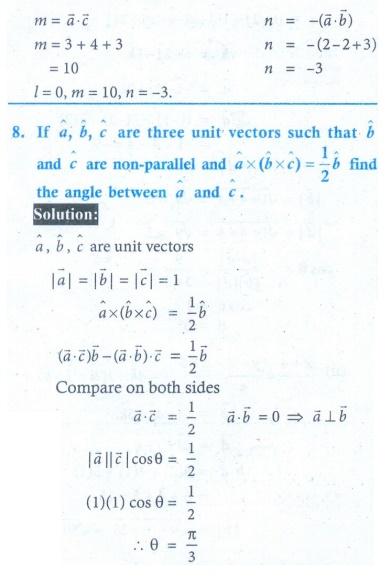Home | | Maths 12th Std | Exercise 6.3: Vector triple product, Jacobi’s Identity and Lagrange’s Identity

# Exercise 6.3: Vector triple product, Jacobi’s Identity and Lagrange’s Identity

Maths Book back answers and solution for Exercise questions - Mathematics : Applications of Vector Algebra: Vector triple product, Jacobi’s Identity and Lagrange’s Identity

EXERCISE 6.3EXERCISE 6.31. (i) − 2 ˆi + 14 ˆj -  22 ˆk  (ii) 22 ˆi + 14 ˆj + 2 ˆk

5. −74

7. l = 0 , m =10 , n = −3

8. θ = π/3

Tags : Problem Questions with Answer, Solution , 12th Mathematics : UNIT 6 : Applications of Vector Algebra
Study Material, Lecturing Notes, Assignment, Reference, Wiki description explanation, brief detail
12th Mathematics : UNIT 6 : Applications of Vector Algebra : Exercise 6.3: Vector triple product, Jacobi’s Identity and Lagrange’s Identity | Problem Questions with Answer, Solution